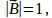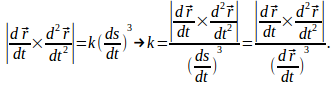## An Equation for the Curvature of a Curve in Terms of Derivatives

Theorem

If a curve in space is given as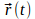and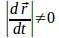then the curvature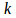is given by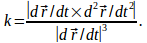Proof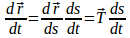hence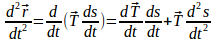Then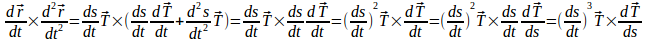since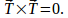Since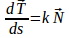and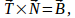the binormal vector, we have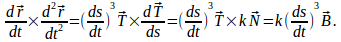Since